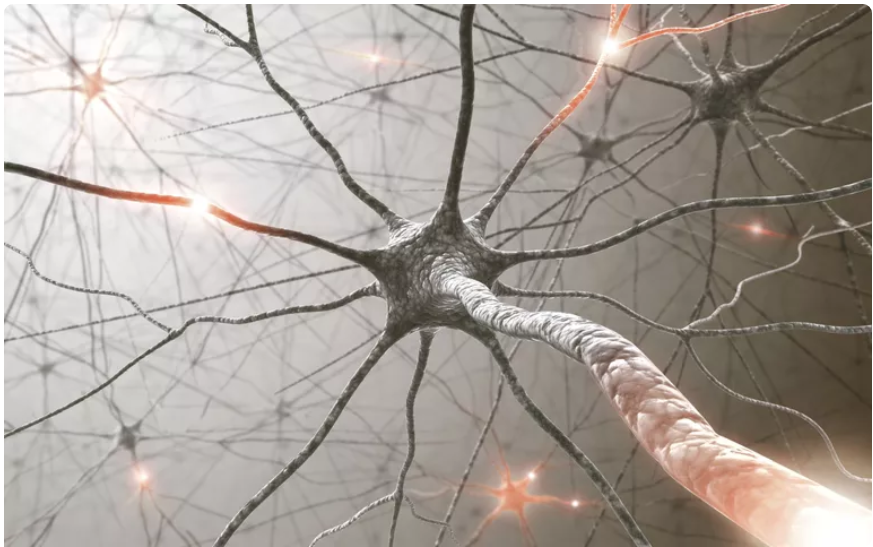# Neuron in a Machine

Original article was published by Swaroop Rajendra on Artificial Intelligence on Medium# Understanding Mathematics behind the working of neurons in Artificial Intelligence.

Let’s assume the probability of neuron passing signal further to outgoing neurons as “P”. Then the probability of neuron, not passing the signal will be “(1-P)”. People, on the other hand, call out the word called “Odds”. i.e., What are the odds if the neuron is going to pass on further to outgoing neurons.

Odds in mathematical terms can be represented as P/(1-P) i.e., the ratio of the probability of a neuron going to pass the signal to the probability of neuron not going to pass the signal.

If we apply log to the above equation, then we can represent the above equation as below:

We know that a neuron receives signals from many incoming neurons. These signals will have values and weights. All the incoming signals are summed up and the summed up value becomes the outgoing signal. Let us assume there are “n” number of incoming neurons connected to a neuron and “x” is the value of signal coming from the incoming neuron and “w” is the weight associated with the signal. It is to remember that if the weight is large (either positive or negative), then it has a strong influence on the outcome. If the weight is small(near to zero), then it has a weak influence on the neuron. If we assume “y” as an outcome or outgoing signal from the neuron, then “y” is nothing but the summed up values of all the incoming signals from all the incoming neurons. Hence we can represent “y” as an equation as shown below:

Summed up values of all the incoming signals can also be represented as shown below. Where W raised to T is the transpose of all the incoming weights.

When the variable we are trying to predict is restricted to have a value between a certain range i.e., 0–1, building such models is challenging. To solve this challenge, we transform these variables into another set of variables that are not restricted to have values in a certain range, and hence logodds come into the picture. The value of log(P/(1-P)) can be between minus infinity to plus infinity. log(P/(1-P)) can be expressed as shown below

logodds function can also be represented in the below form:

If we apply inverse to the above logodds function then it is called the logistic function. This logistic function gives the probability of the outcome of the neuron will be 1 or 0 i.e., will the neuron pass the signal further along or not.

For now, let us assume W raised to T equal to Z as shown below:

Then we can re-write the logodds function like below:

If we apply inverse to the above logodds function to get the logistic function, then we get the below equation:

As said earlier, the output of the above logistic function is nothing but the probability “P”. If we plot the logistic function, it takes the form of the S curve or sigmoid curve. One end of the curve touches 0 at minus infinity and the other end touches 1 at plus infinity.

From the above, we were able to understand how logistic function is derived and how it works. Now let put all our above learning in a model form to understand how a neuron works.